Friday , March 22 2019
Home / Simple Interest | Tips & Tricks# Simple Interest | Tips & Tricks

### Simple Interest Tips, Tricks, and Results

• If the interest on a sum of money is 1/x  of the principal, and the number of years is equal to the rate of interest then rate can be calculated using the formula: √(100/x)

Derivation for this result:
We Know SI = {(P x R x T)/100}
Put SI = P/x  ; and T=R
P/x = {(P x R x T)/100}
R2 = 100/x
R = √(100/x)

Example : The interest on a sum of money is 1/16 of the principal, and the number of years is equal to the rate of interest. What is the rate percent?

Solution :

Using the above concept :

R = √(100/x)

R = √(100/16)

R = 5/2%

### Simple Interest Tips, Tricks and Results

• The rate of interest for t1 years is r1% , t2 years is r1%, tyears is r3 %.If a man gets interest of Rs x for (t1+ t2 + t3 =n)  years, then principal is given by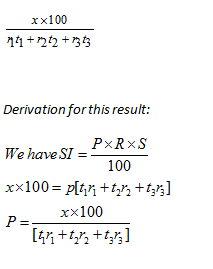Example: The rate of interest for 3 years is 4%, 5years is 6%,1 years is 5%. If a man gets interest of Rs. 4700 for 9 years, calculate the principal amount?Example: A sum of money becomes 4 times in 20 years. Calculate the rate of interest.Example: A sum of money becomes 4 times in 20 years. Calculate the rate of interest.

Solution:

Using the above result:

R = [{100(x-1)}/n]%

R = [{100(4-1)}/20]=15%

### Simple Interest Tips, Tricks, and Results

If a sum of money becomes x times in n years at simple rate of interest, then the time is calculated as

n= {100(x-1)/R}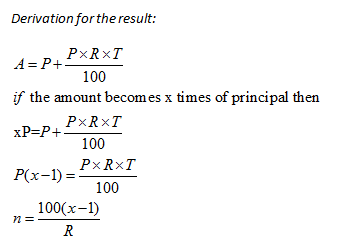Example:A sum was put at SI at a certain rate for 3 years. If the sum would have put at a 2% higher rate, it would have yieldedRs 900 more. What will be the principal?

Solution:

Use the above formula:

P= {100X/(nX r)}

P= {(900 x 100)/(3 x2)} = Rs. 15000

This tooltip is a reverse of the last one. A sum is put at SI at a certain rate for n years. If the sum would have put at a r% lower rate, it could yield Rs X less. So the principal will be:

P= {100X/(n * r)}

Example: A sum was put at SI at a certain rate for 3 years. If the sum would have put at a 2% lower rate, it could yield Rs 900 less. What will be the principal?

Solution:

Using the formula, we arrive at the following:

P= {100X/(n * r)}

P= {(900*100)/(3*2)}

P = Rs. 15000

### Simple Interest Tips, Tricks, and Results

• Change in Simple Interest when time changes from Tto T2 is given by the formula: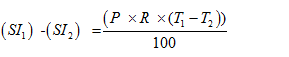• Change in Simple Interest when principal changes from Pto P2  is given by the formula: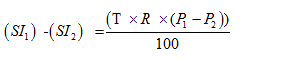• Change in Simple Interest When rate of interest changes from r1to r2 is given by the formula: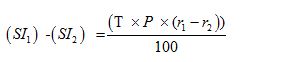Example: Simple interest on Rs 200 increases by Rs 50 when time increases by 5 years. Find rate percent per annum.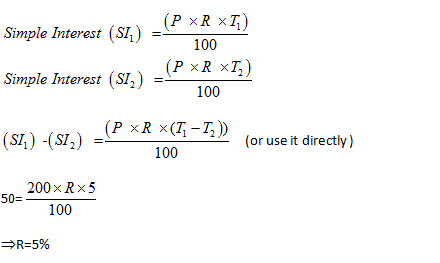Example: If the SI on Rs 1000 be more than the interest on 2000 by Rs 20 in 4 years, find the rate per annum.

Solution: When principal changes from  P1to P2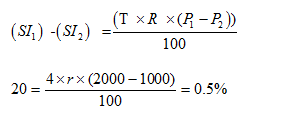### Simple Interest Tips, Tricks, and Results

If a person deposits A1  amount at r1% per annum and amount Aat r2% per annum, then the rate of interest for the whole sum is {(A1r1 + A2r2)/(A1+A2)}

Example: A man deposits Rs.1000 at 4% per annum and Rs 2000 at 5% per annum, find the rate of interest for the whole sum.

Solution:
Method-1:
Using the formula for, Simple Interest (SI) =  {(P x R x T)/100}
X = {(1000 x 4 x 1)/100} = 40
Y = (2000 x 5 x 1)/100 = 100
Total interest = 100+40=Rs. 140
Total principal =1000+2000=Rs.3000
Using the formula:
140 = {(3000 x R x 1)/100}
R = 14/3%

Method-2:
Applying above concept {(A1r1 + A2r2)/(A1+A2)} , the rate of interest is
[{(1000 x 4) + (2000 x 5)}/(1000 + 2000)] = 14000/3000
14/3%

#### A certain amount is invested for certain time but at different rates. When rate per annum is r1 the amount becomes x1 but at rate  r2 per annum, the amount becomes x2 . Time, t, is given by the formula: t = {(x1 – x2)/(x2r1 – x1r2)}*100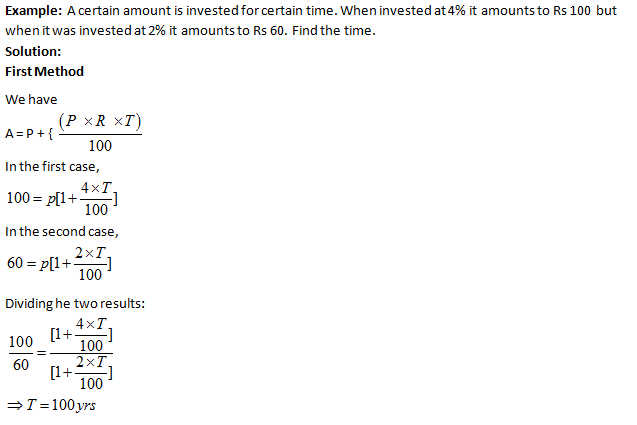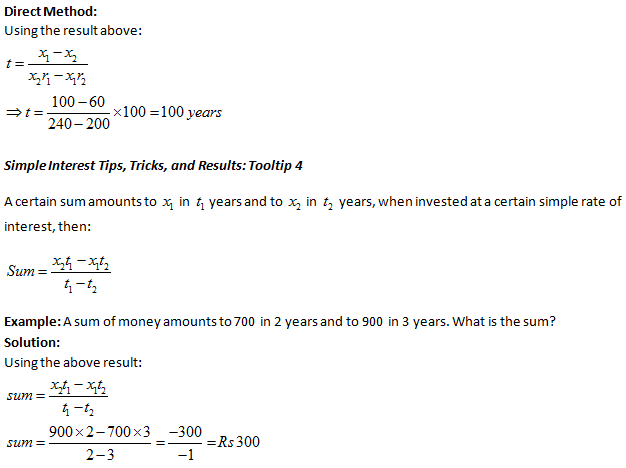error: Content is protected !!